# PEMDAS Explained

Please Excuse My Dear Aunt Sally, or PEMDAS, is a way to remember the order of operations in math. Because so much of mathematics depends on getting the order of operations correct, it’s essential to understand the PEMDAS rules inside and out!

But what is PEMDAS? And what did Aunt Sally do that needs to be excused anyway?Sally knows her order of operations. She has no need for your silly excuses!

## The PEMDAS Rules

Let’s talk about what those six letters stand for.

• P is for parentheses (or brackets, or any other grouping symbol).
• E is for exponents (or things such as roots and radical expressions that are equivalent to exponents).
• MD (do multiplications and divisions left-to-right in the same step).
• M is for multiplication.
• D is for division.
• AS (do additions and subtractions left-to-right in the same step).
• S is for subtraction.The PEMDAS rules specify which operations have priority.

Image by Aha-Soft

For example, let’s work out 7 + 4 × 52.

There are no parentheses (P), so first work out the exponent (E).

7 + 4 × 25

Next, you have to multiply (M).

7 + 100

Finally, the only operation left to do is to add (A).

107

Ta-Da!!! Not bad, right? Well things can get complicated, so let’s explore some of the tricky cases in detail.

### The Left-to-Right Rules

The rules are not quite as simple as they may seem at first. You see, the acronym PEMDAS should really be written more like this: P-E-MD-AS.

• Multiplication (M) and division (D) have equal priority. You have to do all multiplications and divisions from left to right.
• Addition (A) and subtraction (S) also have equal priority. Do all additions and subtractions from left to right in the expression.

For example, to work out 8 — 5 + 4, first subtract (because that’s the leftmost operation), then add.

8 — 5 + 4 = 3 + 4 = 7

If you didn’t follow the correct order of operations, you might get 8 – 9 = -1 instead! So if you mistakenly thought that addition must always come before subtraction because A comes before S in PEMDAS, unfortunately you’d get a lot of problems wrong.

### Ambiguity in Multiplication and Division

The left-to-right rule works exactly the same way for multiplication and division. However, because there are so many different ways to write multiplications and divisions, it can get very confusing. It gets even worse when variables are introduced.

The product of a and b can be written in any of the following ways:

a × b = a × b = ab = (a)b = a(b) = (a)(b)

Similarly, division can be written in-line — that is, not as a vertical fraction — in two ways:

a ÷ b = a/b

Regardless which notations show up, the PEMDAS rules should still work the same way.

For example, 12 ÷ 3 × 2 = 4 × 2 = 8 (MD left-to-right, implies you have to do the division first in this problem). A good way to make sure you’re doing it right is to put in extra parentheses to indicate the grouping explicitly.

12 ÷ 3 × 2 = (12 ÷ 3) × 2 = 4 × 2 = 8

Now let’s try that grouping trick to show how each of the following equivalent expressions works out the same way. Remember, each time we have to do the division first, because it occurs to the left of the multiplication!

• 12/3 × 2 = (12/3) × 2 = 4 × 2 = 8
• 12 ÷ (3)(2) = [12 ÷ (3)](2) = 4(2) = 8
• 12/3x, where x = 2, (12/3)x = 4x = 4(2) = 8

Please Note: if you’re about to write a nasty comment below explaining how wrong I am about 12/3x, please bear with me! These rules are based on current accepted practice. I didn’t make this stuff up. And I guarantee that if you see something like this on the SAT or ACT, then you better believe that they handle it according to the way I’ve explained above!

### The Trouble with Fraction Bars

Caution: There’s a huge difference between 12/(3x) and 12/3x. Endless frustration can occur for students who mix these things up.

Without parentheses, PEMDAS rules imply that you must do division first.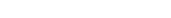With parentheses, the 3x now becomes a group. Multiplication technically must occur before division (but you can still do algebraic simplifications, like cancelling a common factor).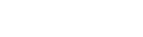### Parentheses and Grouping

The P rule is more like a change-the-rules kind of rule. Parentheses can change the order of operations of an expression because they force some things to be done before others.

For example, consider 5 × (18 — 23).

1. Work out the parentheses, before the multiplication by 5, because P comes before M in PEMDAS.
2. Now inside the parentheses, you have to do the exponent before the subtraction (E before S). This takes us to: 5 × (18 — 8).
3. Next (still within the parentheses), subtract: 5 × (10).
4. Finally, complete the problem by multiplying to get 50.

If you just list out the operations that we did in this problem, you’d get: P->E->S->M. Although it may seem like we broke a rule (shouldn’t M come before S??), we just followed what the P-rule required.

Always think of parentheses (), brackets [], braces {}, and expressions grouped within a radical or on the top or bottom of a fraction bar as a single group. Each single group then needs to be worked out using PEMDAS just within that group.

Let’s see how this works in a more complicated example.

### A Not-So-Easy Example

Simplify: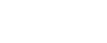When there are multiple groupings, always work inside to out. Find the innermost grouping, use the PEMDAS rules within that group, and then reassess the expression.

First of all, the big fraction bar (called a vinculum; there, that’s a thing you know now) actually serves to group the numerator and denominator into their own separate expressions.

Furthermore, the radical expression acts like a big set of parentheses for the stuff inside of it.

So in a way, we have to think about the P-rule even though no parentheses are present at all (except surrounding the 4, but that just stands for multiplication in this case)!

Let’s start within the radical. 33 — 2(4) = 33 — 8 = 25 (M before S).

Then, simplify the denominator: 2 + 90 = 2 + 1 = 3 (E before A).

Your expression should now look like this: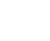Now, we still have to treat the numerator as its own single expression. There is an exponent, a multiplication, and a radical. You have to do the exponent and radical first (E before M).

Don’t forget to simplify the fraction as your last step!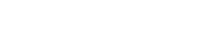## Order of Operations and Algebraic Identities

I want to finish this article with one of my favorite topics, algebraic identities! No really!! I love algebraic identities because they can seemingly allow us to bend the rules about order of operations.

For example, consider the Distributive Identity (or Property, or Law):

a(b + c) = ab + ac

This rule allows you to change a product (of a and (b + c)) into a sum of simpler products that evaluates to the same amount.

Suppose you need to simplify 6(x + 7). Well, by the PEMDAS rules, we should work out what’s in the parentheses first. But I don’t know what x is, and there’s no way that I can add 7 to an unknown amount, right?

However, using the Distributive Identity, I can write:

6(x + 7) = 6x + 6(7)

Now, the order of operations imply that I have to multiply before adding. I still don’t know x, so there’s nothing to do for the 6x term. On the other hand, I do know 6(7) = 42. So we end up with the following equivalent expression.

6x + 42

It may just seem like a useless “trick” at this point, but you’ll find that a lot of algebra depends on changing the order of operations using algebraic identities.

## Conclusion

Just to recap, the PEMDAS rules give the proper order of operations for simplifying mathematical expressions.

• P stands for any kinds of grouping, including parentheses, brackets, and braces, as well as groups that are implied by radical and fractional expressions. Work out all groupings from inside to out.
• E stands for both exponents and radicals.
• MD means that multiplications and divisions must be done from left to right. Be especially careful when there are variables and alternate notations for products and quotients.
• AS means that additions and subtractions must be done last, from left to right.

So, the next time you come across one of those famous “99% can’t solve this” memes involving order of operations on the internet, you can now impress (or annoy) your friends by explaining why they’re all wrong.

By the way, here’s a really informative article that helps to explain why there’s such confusion over seemingly simple mathematical operations. In fact, the PEMDAS rules are just the current conventions for working out complicated multi-operation expressions. Years ago, the rules were actually a bit different. Who knows whether the rules might change again a hundred years from now?

Speaking of the internet, check 8 of the Best YouTube Math Videos for Review for a wealth of information all about math!

## Author

•Shaun earned his Ph. D. in mathematics from The Ohio State University in 2008 (Go Bucks!!). He received his BA in Mathematics with a minor in computer science from Oberlin College in 2002. In addition, Shaun earned a B. Mus. from the Oberlin Conservatory in the same year, with a major in music composition. Shaun still loves music -- almost as much as math! -- and he (thinks he) can play piano, guitar, and bass. Shaun has taught and tutored students in mathematics for about a decade, and hopes his experience can help you to succeed!